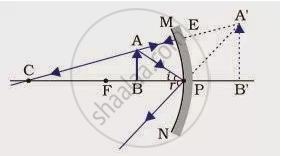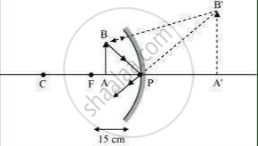Share
Notifications

View all notifications
Books Shortlist
Your shortlist is empty

# We Wish to Obtain an Erect Image of an Object, Using a Concave Mirror of Focal Length 15 Cm. What Should Be the Range of Distance of the Object from the Mirror? - CBSE Class 10 - Science

Login
Create free account

Forgot password?
ConceptRepresentation of Images Formed by Spherical Mirrors Using Ray Diagrams - Concave Mirror

#### Question

We wish to obtain an erect image of an object, using a concave mirror of focal length 15 cm. What should be the range of distance of the object from the mirror? What is the nature of the image? Is the image larger or smaller than the object? Draw a ray diagram to show the image formation in this case.

#### Solution 1

Range of the distance of the object = 0 to 15 cm from the pole of the mirror.
Nature of the image = virtual, erect and larger than the object.#### Solution 2

Range of object distance = 0 cm to15 cm
A concave mirror gives an erect image when an object is placed between its pole (P) and the principal focus (F).
Hence, to obtain an erect image of an object from a concave mirror of focal length 15 cm, the object must be placed anywhere between the pole and the focus. The image formed will be virtual, erect, and magnified in nature, as shown in the given figure.Is there an error in this question or solution?

#### APPEARS IN

NCERT Solution for Science Textbook for Class 10 (2019 to Current)
Chapter 10: Light – Reflection and Refraction
Q: 7 | Page no. 186
Solution We Wish to Obtain an Erect Image of an Object, Using a Concave Mirror of Focal Length 15 Cm. What Should Be the Range of Distance of the Object from the Mirror? Concept: Representation of Images Formed by Spherical Mirrors Using Ray Diagrams - Concave Mirror.
S